# 4.3. Preprocessing data¶

The `sklearn.preprocessing` package provides several common utility functions and transformer classes to change raw feature vectors into a representation that is more suitable for the downstream estimators.

## 4.3.1. Standardization, or mean removal and variance scaling¶

Standardization of datasets is a common requirement for many machine learning estimators implemented in the scikit; they might behave badly if the individual features do not more or less look like standard normally distributed data: Gaussian with zero mean and unit variance.

In practice we often ignore the shape of the distribution and just transform the data to center it by removing the mean value of each feature, then scale it by dividing non-constant features by their standard deviation.

For instance, many elements used in the objective function of a learning algorithm (such as the RBF kernel of Support Vector Machines or the l1 and l2 regularizers of linear models) assume that all features are centered around zero and have variance in the same order. If a feature has a variance that is orders of magnitude larger that others, it might dominate the objective function and make the estimator unable to learn from other features correctly as expected.

The function `scale` provides a quick and easy way to perform this operation on a single array-like dataset:

```>>> from sklearn import preprocessing
>>> import numpy as np
>>> X = np.array([[ 1., -1.,  2.],
...               [ 2.,  0.,  0.],
...               [ 0.,  1., -1.]])
>>> X_scaled = preprocessing.scale(X)

>>> X_scaled
array([[ 0.  ..., -1.22...,  1.33...],
[ 1.22...,  0.  ..., -0.26...],
[-1.22...,  1.22..., -1.06...]])
```

Scaled data has zero mean and unit variance:

```>>> X_scaled.mean(axis=0)
array([ 0.,  0.,  0.])

>>> X_scaled.std(axis=0)
array([ 1.,  1.,  1.])
```

The `preprocessing` module further provides a utility class `StandardScaler` that implements the `Transformer` API to compute the mean and standard deviation on a training set so as to be able to later reapply the same transformation on the testing set. This class is hence suitable for use in the early steps of a `sklearn.pipeline.Pipeline`:

```>>> scaler = preprocessing.StandardScaler().fit(X)
>>> scaler
StandardScaler(copy=True, with_mean=True, with_std=True)

>>> scaler.mean_
array([ 1. ...,  0. ...,  0.33...])

>>> scaler.scale_
array([ 0.81...,  0.81...,  1.24...])

>>> scaler.transform(X)
array([[ 0.  ..., -1.22...,  1.33...],
[ 1.22...,  0.  ..., -0.26...],
[-1.22...,  1.22..., -1.06...]])
```

The scaler instance can then be used on new data to transform it the same way it did on the training set:

```>>> scaler.transform([[-1.,  1., 0.]])
array([[-2.44...,  1.22..., -0.26...]])
```

It is possible to disable either centering or scaling by either passing `with_mean=False` or `with_std=False` to the constructor of `StandardScaler`.

### 4.3.1.1. Scaling features to a range¶

An alternative standardization is scaling features to lie between a given minimum and maximum value, often between zero and one, or so that the maximum absolute value of each feature is scaled to unit size. This can be achieved using `MinMaxScaler` or `MaxAbsScaler`, respectively.

The motivation to use this scaling include robustness to very small standard deviations of features and preserving zero entries in sparse data.

Here is an example to scale a toy data matrix to the `[0, 1]` range:

```>>> X_train = np.array([[ 1., -1.,  2.],
...                     [ 2.,  0.,  0.],
...                     [ 0.,  1., -1.]])
...
>>> min_max_scaler = preprocessing.MinMaxScaler()
>>> X_train_minmax = min_max_scaler.fit_transform(X_train)
>>> X_train_minmax
array([[ 0.5       ,  0.        ,  1.        ],
[ 1.        ,  0.5       ,  0.33333333],
[ 0.        ,  1.        ,  0.        ]])
```

The same instance of the transformer can then be applied to some new test data unseen during the fit call: the same scaling and shifting operations will be applied to be consistent with the transformation performed on the train data:

```>>> X_test = np.array([[ -3., -1.,  4.]])
>>> X_test_minmax = min_max_scaler.transform(X_test)
>>> X_test_minmax
array([[-1.5       ,  0.        ,  1.66666667]])
```

It is possible to introspect the scaler attributes to find about the exact nature of the transformation learned on the training data:

```>>> min_max_scaler.scale_
array([ 0.5       ,  0.5       ,  0.33...])

>>> min_max_scaler.min_
array([ 0.        ,  0.5       ,  0.33...])
```

If `MinMaxScaler` is given an explicit `feature_range=(min, max)` the full formula is:

```X_std = (X - X.min(axis=0)) / (X.max(axis=0) - X.min(axis=0))

X_scaled = X_std / (max - min) + min
```

`MaxAbsScaler` works in a very similar fashion, but scales in a way that the training data lies within the range `[-1, 1]` by dividing through the largest maximum value in each feature. It is meant for data that is already centered at zero or sparse data.

Here is how to use the toy data from the previous example with this scaler:

```>>> X_train = np.array([[ 1., -1.,  2.],
...                     [ 2.,  0.,  0.],
...                     [ 0.,  1., -1.]])
...
>>> max_abs_scaler = preprocessing.MaxAbsScaler()
>>> X_train_maxabs = max_abs_scaler.fit_transform(X_train)
>>> X_train_maxabs                # doctest +NORMALIZE_WHITESPACE^
array([[ 0.5, -1. ,  1. ],
[ 1. ,  0. ,  0. ],
[ 0. ,  1. , -0.5]])
>>> X_test = np.array([[ -3., -1.,  4.]])
>>> X_test_maxabs = max_abs_scaler.transform(X_test)
>>> X_test_maxabs
array([[-1.5, -1. ,  2. ]])
>>> max_abs_scaler.scale_
array([ 2.,  1.,  2.])
```

As with `scale`, the module further provides convenience functions `minmax_scale` and `maxabs_scale` if you don’t want to create an object.

### 4.3.1.2. Scaling sparse data¶

Centering sparse data would destroy the sparseness structure in the data, and thus rarely is a sensible thing to do. However, it can make sense to scale sparse inputs, especially if features are on different scales.

`MaxAbsScaler` and `maxabs_scale` were specifically designed for scaling sparse data, and are the recommended way to go about this. However, `scale` and `StandardScaler` can accept `scipy.sparse` matrices as input, as long as `with_centering=False` is explicitly passed to the constructor. Otherwise a `ValueError` will be raised as silently centering would break the sparsity and would often crash the execution by allocating excessive amounts of memory unintentionally. `RobustScaler` cannot be fited to sparse inputs, but you can use the `transform` method on sparse inputs.

Note that the scalers accept both Compressed Sparse Rows and Compressed Sparse Columns format (see `scipy.sparse.csr_matrix` and `scipy.sparse.csc_matrix`). Any other sparse input will be converted to the Compressed Sparse Rows representation. To avoid unnecessary memory copies, it is recommended to choose the CSR or CSC representation upstream.

Finally, if the centered data is expected to be small enough, explicitly converting the input to an array using the `toarray` method of sparse matrices is another option.

### 4.3.1.3. Scaling data with outliers¶

If your data contains many outliers, scaling using the mean and variance of the data is likely to not work very well. In these cases, you can use `robust_scale` and `RobustScaler` as drop-in replacements instead. They use more robust estimates for the center and range of your data.

References:

Further discussion on the importance of centering and scaling data is available on this FAQ: Should I normalize/standardize/rescale the data?

Scaling vs Whitening

It is sometimes not enough to center and scale the features independently, since a downstream model can further make some assumption on the linear independence of the features.

To address this issue you can use `sklearn.decomposition.PCA` or `sklearn.decomposition.RandomizedPCA` with `whiten=True` to further remove the linear correlation across features.

Scaling target variables in regression

`scale` and `StandardScaler` work out-of-the-box with 1d arrays. This is very useful for scaling the target / response variables used for regression.

### 4.3.1.4. Centering kernel matrices¶

If you have a kernel matrix of a kernel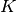that computes a dot product in a feature space defined by function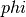, a `KernelCenterer` can transform the kernel matrix so that it contains inner products in the feature space defined byfollowed by removal of the mean in that space.

## 4.3.2. Normalization¶

Normalization is the process of scaling individual samples to have unit norm. This process can be useful if you plan to use a quadratic form such as the dot-product or any other kernel to quantify the similarity of any pair of samples.

This assumption is the base of the Vector Space Model often used in text classification and clustering contexts.

The function `normalize` provides a quick and easy way to perform this operation on a single array-like dataset, either using the `l1` or `l2` norms:

```>>> X = [[ 1., -1.,  2.],
...      [ 2.,  0.,  0.],
...      [ 0.,  1., -1.]]
>>> X_normalized = preprocessing.normalize(X, norm='l2')

>>> X_normalized
array([[ 0.40..., -0.40...,  0.81...],
[ 1.  ...,  0.  ...,  0.  ...],
[ 0.  ...,  0.70..., -0.70...]])
```

The `preprocessing` module further provides a utility class `Normalizer` that implements the same operation using the `Transformer` API (even though the `fit` method is useless in this case: the class is stateless as this operation treats samples independently).

This class is hence suitable for use in the early steps of a `sklearn.pipeline.Pipeline`:

```>>> normalizer = preprocessing.Normalizer().fit(X)  # fit does nothing
>>> normalizer
Normalizer(copy=True, norm='l2')
```

The normalizer instance can then be used on sample vectors as any transformer:

```>>> normalizer.transform(X)
array([[ 0.40..., -0.40...,  0.81...],
[ 1.  ...,  0.  ...,  0.  ...],
[ 0.  ...,  0.70..., -0.70...]])

>>> normalizer.transform([[-1.,  1., 0.]])
array([[-0.70...,  0.70...,  0.  ...]])
```

Sparse input

`normalize` and `Normalizer` accept both dense array-like and sparse matrices from scipy.sparse as input.

For sparse input the data is converted to the Compressed Sparse Rows representation (see `scipy.sparse.csr_matrix`) before being fed to efficient Cython routines. To avoid unnecessary memory copies, it is recommended to choose the CSR representation upstream.

## 4.3.3. Binarization¶

### 4.3.3.1. Feature binarization¶

Feature binarization is the process of thresholding numerical features to get boolean values. This can be useful for downstream probabilistic estimators that make assumption that the input data is distributed according to a multi-variate Bernoulli distribution. For instance, this is the case for the `sklearn.neural_network.BernoulliRBM`.

It is also common among the text processing community to use binary feature values (probably to simplify the probabilistic reasoning) even if normalized counts (a.k.a. term frequencies) or TF-IDF valued features often perform slightly better in practice.

As for the `Normalizer`, the utility class `Binarizer` is meant to be used in the early stages of `sklearn.pipeline.Pipeline`. The `fit` method does nothing as each sample is treated independently of others:

```>>> X = [[ 1., -1.,  2.],
...      [ 2.,  0.,  0.],
...      [ 0.,  1., -1.]]

>>> binarizer = preprocessing.Binarizer().fit(X)  # fit does nothing
>>> binarizer
Binarizer(copy=True, threshold=0.0)

>>> binarizer.transform(X)
array([[ 1.,  0.,  1.],
[ 1.,  0.,  0.],
[ 0.,  1.,  0.]])
```

It is possible to adjust the threshold of the binarizer:

```>>> binarizer = preprocessing.Binarizer(threshold=1.1)
>>> binarizer.transform(X)
array([[ 0.,  0.,  1.],
[ 1.,  0.,  0.],
[ 0.,  0.,  0.]])
```

As for the `StandardScaler` and `Normalizer` classes, the preprocessing module provides a companion function `binarize` to be used when the transformer API is not necessary.

Sparse input

`binarize` and `Binarizer` accept both dense array-like and sparse matrices from scipy.sparse as input.

For sparse input the data is converted to the Compressed Sparse Rows representation (see `scipy.sparse.csr_matrix`). To avoid unnecessary memory copies, it is recommended to choose the CSR representation upstream.

## 4.3.4. Encoding categorical features¶

Often features are not given as continuous values but categorical. For example a person could have features `["male", "female"]`, `["from Europe", "from US", "from Asia"]`, `["uses Firefox", "uses Chrome", "uses Safari", "uses Internet Explorer"]`. Such features can be efficiently coded as integers, for instance `["male", "from US", "uses Internet Explorer"]` could be expressed as `[0, 1, 3]` while `["female", "from Asia", "uses Chrome"]` would be `[1, 2, 1]`.

Such integer representation can not be used directly with scikit-learn estimators, as these expect continuous input, and would interpret the categories as being ordered, which is often not desired (i.e. the set of browsers was ordered arbitrarily).

One possibility to convert categorical features to features that can be used with scikit-learn estimators is to use a one-of-K or one-hot encoding, which is implemented in `OneHotEncoder`. This estimator transforms each categorical feature with `m` possible values into `m` binary features, with only one active.

Continuing the example above:

```>>> enc = preprocessing.OneHotEncoder()
>>> enc.fit([[0, 0, 3], [1, 1, 0], [0, 2, 1], [1, 0, 2]])
OneHotEncoder(categorical_features='all', dtype=<... 'float'>,
handle_unknown='error', n_values='auto', sparse=True)
>>> enc.transform([[0, 1, 3]]).toarray()
array([[ 1.,  0.,  0.,  1.,  0.,  0.,  0.,  0.,  1.]])
```

By default, how many values each feature can take is inferred automatically from the dataset. It is possible to specify this explicitly using the parameter `n_values`. There are two genders, three possible continents and four web browsers in our dataset. Then we fit the estimator, and transform a data point. In the result, the first two numbers encode the gender, the next set of three numbers the continent and the last four the web browser.

See Loading features from dicts for categorical features that are represented as a dict, not as integers.

## 4.3.5. Imputation of missing values¶

For various reasons, many real world datasets contain missing values, often encoded as blanks, NaNs or other placeholders. Such datasets however are incompatible with scikit-learn estimators which assume that all values in an array are numerical, and that all have and hold meaning. A basic strategy to use incomplete datasets is to discard entire rows and/or columns containing missing values. However, this comes at the price of losing data which may be valuable (even though incomplete). A better strategy is to impute the missing values, i.e., to infer them from the known part of the data.

The `Imputer` class provides basic strategies for imputing missing values, either using the mean, the median or the most frequent value of the row or column in which the missing values are located. This class also allows for different missing values encodings.

The following snippet demonstrates how to replace missing values, encoded as `np.nan`, using the mean value of the columns (axis 0) that contain the missing values:

```>>> import numpy as np
>>> from sklearn.preprocessing import Imputer
>>> imp = Imputer(missing_values='NaN', strategy='mean', axis=0)
>>> imp.fit([[1, 2], [np.nan, 3], [7, 6]])
Imputer(axis=0, copy=True, missing_values='NaN', strategy='mean', verbose=0)
>>> X = [[np.nan, 2], [6, np.nan], [7, 6]]
>>> print(imp.transform(X))
[[ 4.          2.        ]
[ 6.          3.666...]
[ 7.          6.        ]]
```

The `Imputer` class also supports sparse matrices:

```>>> import scipy.sparse as sp
>>> X = sp.csc_matrix([[1, 2], [0, 3], [7, 6]])
>>> imp = Imputer(missing_values=0, strategy='mean', axis=0)
>>> imp.fit(X)
Imputer(axis=0, copy=True, missing_values=0, strategy='mean', verbose=0)
>>> X_test = sp.csc_matrix([[0, 2], [6, 0], [7, 6]])
>>> print(imp.transform(X_test))
[[ 4.          2.        ]
[ 6.          3.666...]
[ 7.          6.        ]]
```

Note that, here, missing values are encoded by 0 and are thus implicitly stored in the matrix. This format is thus suitable when there are many more missing values than observed values.

`Imputer` can be used in a Pipeline as a way to build a composite estimator that supports imputation. See Imputing missing values before building an estimator

## 4.3.6. Generating polynomial features¶

Often it’s useful to add complexity to the model by considering nonlinear features of the input data. A simple and common method to use is polynomial features, which can get features’ high-order and interaction terms. It is implemented in `PolynomialFeatures`:

```>>> import numpy as np
>>> from sklearn.preprocessing import PolynomialFeatures
>>> X = np.arange(6).reshape(3, 2)
>>> X
array([[0, 1],
[2, 3],
[4, 5]])
>>> poly = PolynomialFeatures(2)
>>> poly.fit_transform(X)
array([[  1.,   0.,   1.,   0.,   0.,   1.],
[  1.,   2.,   3.,   4.,   6.,   9.],
[  1.,   4.,   5.,  16.,  20.,  25.]])
```

The features of X have been transformed from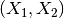to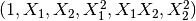.

In some cases, only interaction terms among features are required, and it can be gotten with the setting `interaction_only=True`:

```>>> X = np.arange(9).reshape(3, 3)
>>> X
array([[0, 1, 2],
[3, 4, 5],
[6, 7, 8]])
>>> poly = PolynomialFeatures(degree=3, interaction_only=True)
>>> poly.fit_transform(X)
array([[   1.,    0.,    1.,    2.,    0.,    0.,    2.,    0.],
[   1.,    3.,    4.,    5.,   12.,   15.,   20.,   60.],
[   1.,    6.,    7.,    8.,   42.,   48.,   56.,  336.]])
```

The features of X have been transformed from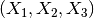to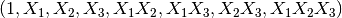.

Note that polynomial features are used implicitily in kernel methods (e.g., `sklearn.svm.SVC`, `sklearn.decomposition.KernelPCA`) when using polynomial Kernel functions.

See Polynomial interpolation for Ridge regression using created polynomial features.

## 4.3.7. Custom transformers¶

Often, you will want to convert an existing Python function into a transformer to assist in data cleaning or processing. You can implement a transformer from an arbitrary function with `FunctionTransformer`. For example, to build a transformer that applies a log transformation in a pipeline, do:

```>>> import numpy as np
>>> from sklearn.preprocessing import FunctionTransformer
>>> transformer = FunctionTransformer(np.log1p)
>>> X = np.array([[0, 1], [2, 3]])
>>> transformer.transform(X)
array([[ 0.        ,  0.69314718],
[ 1.09861229,  1.38629436]])
```

For a full code example that demonstrates using a `FunctionTransformer` to do custom feature selection, see Using FunctionTransformer to select columns